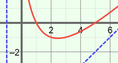# General Form of a Rational Function

### DESCRIPTION

Compare the equation of a rational function to its graph. Multiply or divide the numerator and denominator by linear factors and explore how the graph changes in response.

Full Lesson Info